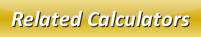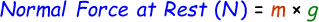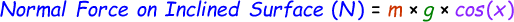# Normal Force Calculator

Normal Force is the force that acts perpendicularly to the surface. It is the quantity of force used for counteracting the gravity.

Formula to calculate normal force at rest or level surface is given by:Formula to calculate normal force on an incline surface is given by:where,
N = Normal Force
m = Mass of the object
g = Acceleration of gravity [9.8m/s2]
x = Angle of Inclination

Enter the input values in our below online normal force calculator and click calculate button to find the answer.

Note: If you're trying to calculate normal force at rest then enter the angle of inclination as zero in the below calculator.

 Mass of the Object [m]: [kg] Acceleration of Gravity (g): [m/s2] Angle of Inclination (x): [degrees] [Enter 0 for calculating normal force on level surface] Normal Force [N]: [N]

Latest Calculator Release

Average Acceleration Calculator

Average acceleration is the object's change in speed for a specific given time period. ...

Free Fall Calculator

When an object falls into the ground due to planet's own gravitational force is known a...

Torque Calculator

Torque is nothing but a rotational force. In other words, the amount of force applied t...

Average Force Calculator

Average force can be explained as the amount of force exerted by the body moving at giv...

Angular Displacement Calculator

Angular displacement is the angle at which an object moves on a circular path. It is de...